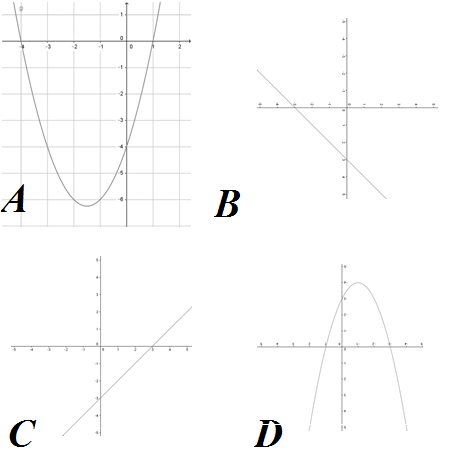##### KAPEC GEOTASK?
• The learning process is entertaining and exciting
• The solved tasks turn into coordinates
• The chance to follow your progress
• Along with your schoolmates and friends, go on an adventure, searching for hideouts
• E-mail:

# 2343.3. Quadratic function y = ax² + bx + c concept

#### Characterise the quadratic function y=x^2+3x-4!What is the coefficient of the quad member?
What is the graphic dispiction of the given function?
What is the branch direction of the function's graph?
Which of the graphic sketches given illustrates the given quadratic function?

#### Next task: 2332. Quadratic function y = ax² + bx + c concept

Grade:
9
Subject
Algebra
Theme
Square function
Subtheme
Quadratic function y = ax² + bx + c concept

#### Solve tasks to find hidden Geo point coordinates